# How to use HLOOKUP in Excel with formula examples

As you probably know, Microsoft Excel has three functions to look up a value - LOOKUP, VLOOKUP and HLOOKUP - and they seem to confuse users the most. In this tutorial, we will focus on the specificities of the Excel HLOOKUP function and discuss a few formula examples that will help you use it in Excel most efficiently.

## What is HLOOKUP in Excel?

The Excel HLOOKUP function is designed for horizontal lookup. More specifically, it searches for a certain value in the first row of the table and returns another value in the same column from a row that you specify.

The HLOOKUP function is available in all versions of Microsoft Excel 2016, Excel 2013, Excel 2010, Excel 2007 and lower.

### Excel HLOOKUP syntax and uses

The HLOOKUP function in Excel has the following arguments:

HLOOKUP(lookup_value, table_array, row_index_num, [range_lookup])
• Lookup_value (required) - the value to search for. It can be a cell reference, numeric value or text string.
• Table_array (required) - two or more rows of data in which the lookup value is searched. It can be a regular range, named range or table. Lookup values should always be located in the first row of table_array.
• Row_index_num (required) - the row number in table_array from which the value should be returned. For example, to return the matching value from the 2nd row, set row_index_num to 2, and so on.
• Range_lookup (optional) - a logical (Boolean) value that instructs HLOOKUP to search with exact or approximate match.

If TRUE or omitted, an approximate match is returned. What it means is if an exact match is not found, your Hlookup formula will do a non-exact match and return the next largest value that is less than lookup_value.

If FALSE, only an exact match is returned. If no value in a specified row matches the lookup value exactly, HLOOKUP throws the #N/A error.

To make things easier to understand, you can translate Excel's HLOOKUP syntax:

HLOOKUP(lookup_value, table_array, row_index_num, [range_lookup])

into normal English:

HLOOKUP(search for this value, in this table, return a value from this row, [return an approximate or exact match])

To see how it works in practice, let's make a simple Hlookup example. Supposing you have a table with some basic information about the planets of our Solar system (please see the screenshot below). What you want is a formula that returns the diameter of the planet whose name is entered in cell B5.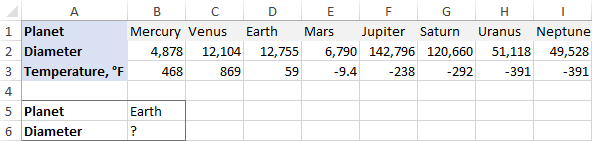In our Hlookup formula, we will be using the following arguments:

• Lookup_value is B5 - the cell containing the planet name you want to find.
• Table_array is B2:I3 - the table where the formula will look up the value.
• Row_index_num is 2 because Diameter is the 2nd row in the table.
• Range_lookup is FALSE. Because the first row of our table is not sorted from A to Z, we can only look up with exact match, which works just fine in this example.

Now you put the arguments together and get the following formula:

`=VLOOKUP(40, A2:B15,2)`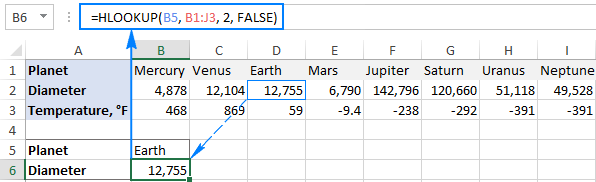### 3 things you should know about Excel HLOOKUP function

Whenever you do a horizontal lookup in Excel, please remember the following facts:

1. The HLOOKUP function can only search in the top-most row of table_array. If you need to look up somewhere else, consider using an Index / Match formula.
2. HLOOKUP in Excel is case-insensitive, it does not distinguish uppercase and lowercase.
3. If range_lookup is set to TRUE or omitted (approximate match), the values in the first row of table_array must be sorted in ascending order (A-Z) left to right.

## What is the difference between VLOOKUP and HLOOKUP in Excel?

As you already know, both functions VLOOKUP and HLOOKUP search for a lookup value. The difference is how the search is performed. As you probably noticed, the functions' names differ only in the first letter - "H" stands for horizontal, and "V" for vertical.

Hence, you use the VLOOKUP function to search is vertical lists when your lookup values are located in a column to the left of the data that you want to find.

The HLOOKUP function performs a horizontal lookup - it searches for a lookup value at the top-most row of the table and returns a value located a specified number of rows down in the same column.

The following image demonstrates the difference between Vlookup and Hlookup formulas in Excel: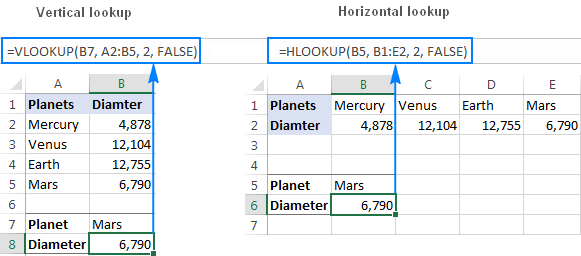## How to use HLOOKUP in Excel - formula examples

Now that the HLOOKUP function has started to look a bit more familiar to you, let's discuss a few more formula examples to consolidate the knowledge.

### Horizontal lookup with approximate and exact match

As you already know, the HLOOKUP function in Excel can perform a lookup with exact and non-exact match depending on which value is supplied to the range_lookup argument:

• TRUE or omitted - approximate match
• FALSE - exact match

Please keep in mind that although we say "approximate match", any Hlookup formula searches for an exact match in the first place. But setting the last argument to FALSE allows the formula to return an approximate match (the nearest value that is less than the lookup value) if an exact match is not found; TRUE or omitted returns the #N/A error in this case.

To better illustrate the point, consider the following HLOOKUP examples.

#### HLOOKUP with approximate match

Supposing you have a list of planets in row 2 (B2:I2) and their temperatures in row 1(B1:I1). You want to find out which planet has a certain temperature that is input in cell B4.

You cannot rely on the off chance that your users know the lookup temperature exactly, so it makes sense to return a nearest match if an exact value in not found.

For instance, to find out the planet whose average temperature is around -340 °F, use the following formula (range_lookup set to TRUE or omitted as in this example):

`=HLOOKUP(B4, B1:I2, 2)`

Please remember that an approximate match requires sorting the values in the top row from smallest to largest or from A to Z, otherwise your Hlookup formula may return a wrong result.

As you can see in the screenshot below, our formula returns Uranus, one of the coldest planets in the Solar System maintaining an average of -346 degrees Fahrenheit.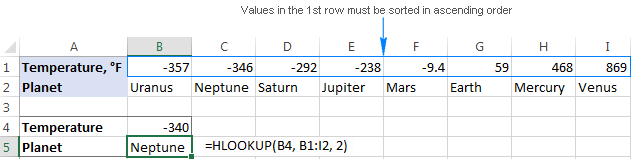#### HLOOKUP with exact match

If you know the lookup value exactly, you can set the last parameter of HLOOKUP to FALSE:

`=HLOOKUP(B4, B1:I2, 2, FALSE)`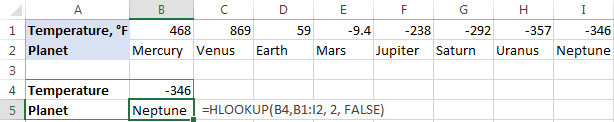On the one hand, an approximate match Hlookup is more user-friendly because it does not require sorting data in the first row. On the other hand, if the exact match is not found, an #N/A error will be returned.

Tip. Not to scare your users by N/A errors, you can embed your Hlookup formula in IFERROR and display your own message, for example:

`=IFERROR(HLOOKUP(B4, B1:I2, 2, FALSE), "Sorry, nothing has been found")`

### How to do HLOOKUP from another worksheet or workbook

In general, h-lookup from another sheet or a different workbook means nothing else than supplying external references to your HLOOKUP formula.

To pull out matching data from a different worksheet, you specify the sheet name followed by an exclamation mark. For example:

`=HLOOKUP(B\$1, Diameters!\$B\$1:\$I\$2,2,FALSE)`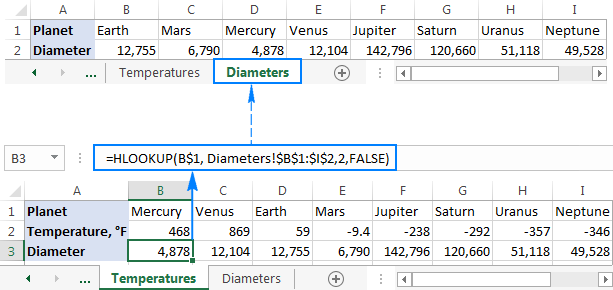If the worksheet name contains spaces or non-alphabetical characters, enclose the name in single quotation marks, like this:

`=HLOOKUP(B\$1, 'Planet diameters'!\$B\$1:\$I\$2,2,FALSE)`

When referencing another workbook, include the workbook name enclosed in square brackets:

`=HLOOKUP(B\$1, [Book1.xlsx]Diameters!\$B\$1:\$I\$2, 2, FALSE)`

If you are pulling data from a closed workbook, the entire path should be specified:

`=HLOOKUP(B\$1, 'D:\Reports\[Book1.xlsx]Diameters'!\$B\$1:\$I\$2, 2, FALSE)`

Tip. Instead of typing the workbook and worksheet names in the formula manually, you can select the cells in another sheet and Excel will add an external reference to your formula automatically.

### Excel HLOOKUP with partial match (wildcard characters)

As is the case with VLOOKUP, Excel's HLOOKUP function allows using the following wildcard characters in the lookup_value argument:

• Question mark (?) to match any single character
• Asterisk (*) to match any sequence of characters

Wildcards come in handy when you want to pull information from a database based on some text that is part of the lookup cell's contents.

For example, you have a list of customer names in row 1 and order IDs in row 2. You want to find the order id for a specific customer but you cannot remember the customer name exactly, though you do remember it begins with "ace".

Assuming your data are in cells B1:I2 (table_array) and order numbers are in row 2 (row_index_num), the formula goes as follows:

`=HLOOKUP("ace*", B1:I2, 2, FALSE)`

To make the formula more flexible, you can type the lookup value in a special cell, say B4, and concatenate that cell with the wildcard character, like this:

`=HLOOKUP(B4&"*", B1:I2, 2, FALSE)`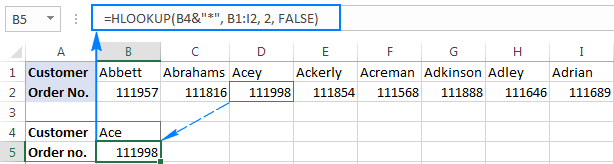Notes.

• For a wildcard HLOOKUP formula to work correctly, the range_lookup argument needs to be set to FALSE.
• If table_array contains more than one value that meets the wildcard criteria, the first found value is returned.

## Absolute and relative cell references in HLOOKUP formulas

If you are writing a formula for a single cell, you may not worry about the proper use of relative and absolute cell references, either one will do.

Copying a formula to multiple cells is a different story. In essence:

• You should always fix table_array by using absolute cell references with the dollar sign (\$) like \$B\$1:\$I\$2.
• Typically, the lookup_value reference is relative or mixed depending on your business logic.

To make things clearer, let's have a closer look at the formula that pulls data from another sheet:

`=HLOOKUP(B\$1, Diameters!\$B\$1:\$I\$2,2,FALSE)`

In the above formula, we use absolute cell references (\$B\$1:\$I\$2) in table_array because it should remain constant when the formula is copied to other cells.

For lookup_value (B\$1), we use a mixed reference, relative column and absolute row, because our lookup values (planet names) are in the same row (row 1) but in different columns (from B to I) and the column reference should change based on a relative position of a cell where the formula in copied.

Due to the clever use of cell references, our Hlookup formula works perfectly for multiple cells: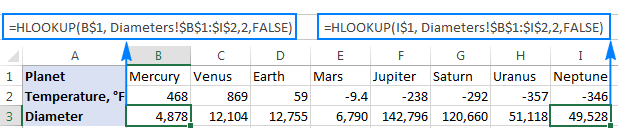## INDEX/MATCH - a more powerful alternative to Excel HLOOKUP

As you already know, the HLOOKUP function in Excel has a number of limitations, the most significant ones are its inability to look up anywhere except for the topmost row, and the necessary to sort values when searching with approximate match.

Luckily, there exists a more powerful and versatile alternative to Vlookup and Hlookup in Excel - the liaison of INDEX and MATCH functions, which boils down to this generic formula:

INDEX (where to return a value from, MATCH (lookup value, where to search, 0))

Assuming your lookup value is in cell B7, you are looking for a match in row 2 (B2:I2), and want to return a value from row 1 (B1:I1), the formula is as follows:

`=INDEX(B1:I1,MATCH(B7,B2:I2,0))`

In the screenshot below, you can see 2 Hlookup formulas that search in the first and second rows, and in both cases INDEX MATCH works equally well.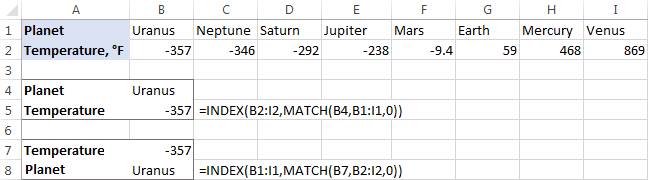For the detailed explanation of the formula's logic and more examples, please see INDEX MATCH as a better alternative to VLOOKUP.

## How to do case-sensitive h-lookup in Excel

As mentioned in the beginning of this tutorial, the Excel HLOOKUP function is case insensitive. In situations when the character case matters, you can take the EXACT function that compares cells exactly, and put it inside the INDEX MATCH formula discussed in the previous example:

INDEX (row to return a value from, MATCH(TRUE, EXACT(row to search in, lookup value), 0))

Assuming your lookup value is in cell B4, the lookup range is B1:I1, and the return range is B2:I2, the formula takes the following shape:

`=INDEX(B2:I2, MATCH(TRUE, EXACT(B1:I1,B4),0))`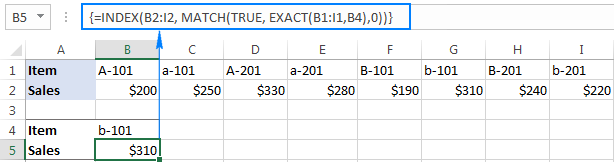Important note! It is an array formula and therefore you should press Ctrl + Shift + Enter to complete it.

The above example demonstrates my favorite but not the only possible way to do a case-sensitive Hlookup in Excel. If you are curious to know other techniques, please check out this tutorial: 4 ways to do a case-sensitive Vlookup in Excel. I think you won't have any problems with rebuilding the Vlookup formulas for a horizontal lookup.

## Top 10 reasons why Excel HLOOKUP is not working

By now you already know that Hlookup is a very useful and powerful lookup function in Excel. It is also a tricky one, and because of its numerous specificities #N/A, #VALUE or #REF errors are a common sight. If your HLOOKUP formula does not work properly, most likely it's because of one of the following reasons.

### 1. HLOOKUP in Excel cannot look above itself

Even if you forget all other details about horizontal lookup in Excel, please remember this essential one - Hlookup can only search in the top-most row of the table. If case your lookup values reside in some other row, an N/A error is returned. To overcome this limitation, use an INDEX MATCH formula.

### 2. Approximate match vs. exact match

When doing a lookup in Excel, either horizontal (Hlookup) or vertical (Vlookup), in most cases you'd be searching for a particular thing, and therefore require an exact match. When searching with approximate match (range_lookup set to TRUE or omitted), remember to sort values in the first row in ascending order.

### 3. The table array reference changes when copying the formula

When using multiple HLOOKUPs to retrieve information about a row of lookup values, you have to lock the table_array reference as demonstrated in Absolute and relative cell references in Hlookup formulas.

### 4. Inserting or deleting a new row

To understand why inserting a new row can break an Hlookup formula, remember how Excel HLOOKUP gets information about the lookup value - based on the row index number you specify.

Supposing, you want to get sales figures based on the product ID. Those figures are in row 4, so you type 4 in the row_index_num argument. But after a new row has been inserted, it becomes row 5... and your Hlookup stops working. The same problem may occur when deleting an existing row from the table.

The solution is either lock the table to prevent your users from inserting new rows, or use INDEX & MATCH instead of Hlookup. In Index/Match formulas, you specify the rows to look up and to return values from as range references, not index numbers, and Excel is smart enough to adjust those references on the fly. So, you are free to delete or insert as many columns and rows as you want without worrying about updating every formula in your worksheet.

### 5. Duplicates in the table

The HLOOKUP function in Excel can only return one value, which is the first value in the table that matches the lookup value.

If there are a few identical records in your table, choose one of the following solutions that best suits your needs:

### 6. Extra spaces

When your obviously correct Hlookup formula returns a bunch of #N/A errors, check your table and lookup value for extra spaces. You can quickly remove leading, trailing and excess in-between spaces by using Excel TRIM function or our Cell Cleaner tool.

### 7. Numbers formatted as text

Text strings that look like numbers are another stumbling block for Excel formulas. The detailed description of this issue and possible solutions are described in Why Excel formulas may stop working.

### 8. Lookup value exceeds 255 characters

All Lookup functions in Excel work only as long as a lookup value is under 255 characters. A longer lookup value results in the #VALUE! error. Since the INDEX /MATCH formula is free of this limitation, use it to overcome this hurdle.

### 9. Full path to the lookup workbook is not specified

If you perform h-lookup from another workbook, remember to supply the full path to it. A couple of formula examples can be found here: How to do Hlookup from another worksheet or workbook.

### 10. Wrong arguments

It has already been highlighted more than once that HLOOKUP is a demanding function that should be treated with great care. Below are a few most common errors caused by supplying incorrect arguments:

• If row_index_num is less than 1, the HLOOKUP function returns the #VALUE! error.
• If row_index_num is greater than the number of rows in table_array, #REF! error is returned.
• If you search with approximate match and your lookup_value is smaller than the smallest value in the first row of table_array, the #N/A error is returned.

Well, this is how to use HLOOKUP in Excel. Hopefully you will find this information helpful. I thank you for reading and hope to see you on our blog next week!

Excel HLOOKUP formula examples

### 25 responses to "How to use HLOOKUP in Excel with formula examples"

1.Anand Kumar says:

Nice........

2.Rohan Singh says:

Thanks a lot for this information. I tried Hlookup with Match function as well and worked very well. Thanks

3.Imran Masud says:

Lot of thanks.

4.gyan says:

plz send excel file

5.Shadab alam says:

While using index match in a range of table having subtotals,the next line below the subtotal fetch the value in subtotal row.Please help?

6.anubhav singh says:

good explain this formulas

7.BONGU MURALIDHARA RAO says:

Good Evening.
I'm very much enthusiastic to get the value of a column C4 with the help of Hlookup
Example. B1 is a merged Column of 3 Colums b2,c2,d2, values are placed in b3,c3,d3 and b4,c4,d4. It it possible with Hlookup, plz explain me

Thanking u

8.josh says:

how can i know that it's using a hlookup formula

9.Ciki says:

With calculation set to automatic, this function will always calculate: =IF(a=b,a,NOW()) a) When F9 is pressed b) When SHIFT + F9 is pressed c) Never as it has no dependents d) When a column is inserted e) When a name is defined on the worksheet

10.Anthony says:

I have an excel sheet with three rows. Each row has a list of usernames I want to hlookup to compare all three rows to match and make a list of the duplicate names. What is the formula that I can use?

•Doug says:

Anthony:
If the data is in three columns Q through S in cells Q1:S10 then in U1 enter:
=IF(SUMPRODUCT((\$Q\$1:\$Q\$10=Q1)*1,(\$R\$1:\$R\$10=R1)*1,(\$S\$1:\$S\$10=S1)*1)>1,"Duplicates","No Duplicates") if this works copy it down the rows.
If the data is in four columns, say Q through T, add a \$T\$1 expression. If there are more than four columns, you can add more expressions, but if you get more than 10 columns I'd rethink the data structure or how I'd approach the problem.

11.NAJJUKO MARIA says:

As Anthony's formula is hard can it be used by A level students of ICT?

12.Vishal says:

how to create conditional dropdown list with the help of hlookup and indirect fuction.

13.Athelstan says:

how to search a single word from a multiple list of data using any of these 3(Hlookup,Vlookup or Lookup?

14.Paul Gardner-Stephen says:

When minimising the add at the bottom of the screen, the blasted thing keeps on scrolling by with an animation, which is VERY distracting. Please get rid of it. I am now off to find a website where this isn't there...

15.hASMUKH says:

If data sheet is in another folder, then how can link it in VLOOKUP or HLOOKUP formulas?

16.MELISSA says:

WHEN USING HLOOKUP, I KEEP GETTING AN ERROR CODE, BECAUSE THE LOOKUP VALUE IS A FORMULA. IS THERE A WAY TO USE THE HLOOKUP FORMULA, WHEN THE LOOKUP VALUE IS A FORMULA?

17.Raj says:

Excellent

18.MJ says:

How do I use an IFBLANK formula with a HLOOKUP formula together so I can essentially use two HLOOKUP's together if my cell is blank?
I have a cell with a date on it and I am using the HLOOKUP to match that date with my data. If that cell is blank I want it to look at a different date and HLOOKUP formula.
Example: =HLOOKUP(Home!\$D\$17,'All Other Measures'!\$B\$2:\$Z\$29,4,0)*(ISBLANK((HLOOKUP(Home!\$D\$18,'All Other Measures'!\$B\$2:\$AL\$29,4,0))))
This formula doesn't work but gives you an idea of what I am trying to achieve.

•Alexander Trifuntov (Ablebits.com Team) says:

Hello!
If I understand your task correctly, the following formula should work for you:

=IF(ISBLANK(Home!\$D\$17), HLOOKUP(Home!\$D\$18,’AllOtherMeasures’!\$B\$2:\$AL\$29,4,0), HLOOKUP(Home!\$D\$17,’AllOtherMeasures’!\$B\$2:\$Z\$29,4,0))

Unfortunately, I can not check its operation, because I do not have your data
I hope this will help

•MJ says:

Thanks for your reply Alexander I really appreciate it. I ended up by changing my formula to an INDEX formula and got it to work perfectly I used this formula:

=IF(INDEX(C5:AL5,MATCH(Home!\$D\$17,'All Other Measures'!\$C\$2:\$AL\$2,0))>0,INDEX(C5:AL5,MATCH(Home!\$D\$17,'All Other Measures'!\$C\$2:\$AL\$2,0)),(INDEX(C5:AL5,MATCH(Home!\$D\$18,'All Other Measures'!\$C\$2:\$AL\$2,0))))

19.Emmanuel Odion says:

=VLOOKUP(D7,A11:J12,1,TRUE) it gives me "N/A" i do not understand,please i need help

•Alexander Trifuntov (Ablebits.com Team) says:

Hello!
20.AR says:
•Alexander Trifuntov (Ablebits.com Team) says: